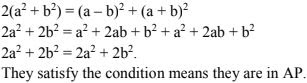# RD Sharma Solutions Chapter 9 Arithmetic Progression Exercise 9.4 Class 10 Maths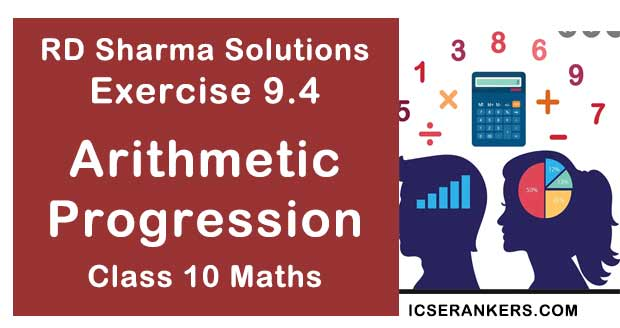Chapter Name RD Sharma Chapter 9 Arithmetic Progression Book Name RD Sharma Mathematics for Class 10 Other Exercises Exercise 9.1Exercise 9.2Exercise 9.3Exercise 9.5MCQ Related Study NCERT Solutions for Class 10 Maths

### Exercise 9.4 Solutions

1. The sum of three terms of an A.P. is 21 and the product of the first and the third terms exceeds the second term by 6, find three terms.

Solution

Given,
Sum of three terms of on A.P. is  21.
Product of first and the third term exceeds the second term by 6.
Let, the three numbers be a -d, a, a +d, with common difference d: then,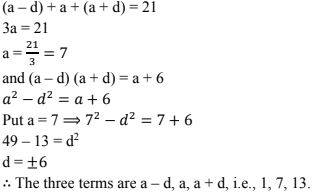2. Three numbers are in A.P.  If the sum of these numbers be 27 and the product 648, find the numbers.

Solution

Let, the three numbers are a - d, a, a +d.
Given,
(a - d) + a + (a + d) = 27
3a = 27
a = 27/3 = 9
and, (a -d )(a) (a + d) = 648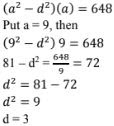∴ The three terms are a - d, a + d i.e., 6,9, 12.

3. Find the four numbers in A.P., whose sum is 50 and in which the greatest number is 4 times the least.

Solution

Let, the four numbers be a - 3d, a - d, a +d, a + 3d, with common difference 2d.
Given, sum is 50.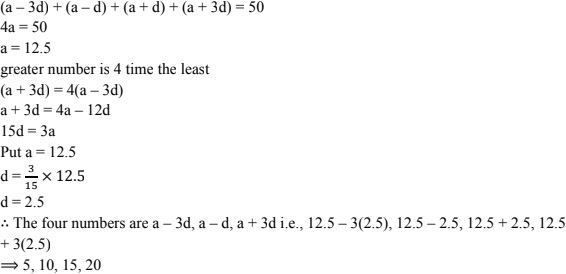4. The angles of a quadrilateral are in A.P. whose common difference is 10°. Find the angles.
Solution
A quadrilateral has four angles. Given, four angles are in A.P. with common difference 10.
Let, the four angles be, a - 3d, a-d, a +d, a + 3d with common difference = 2d.
2d = 10
d = 10/2 = 5
In a quadrilateral, sum of all angles = 360°
(a - 3d) + (a - d) + (a + d) + (a+ 3d) = 360
⇒ 4a = 360
⇒ a = 360/4 = 90°
∴ The angles are a - 3d, a- d, a + d, a+ 3d with a = 90, d = 5
i.e. 90 - 3(5), 90 - 5 , 90 + 5, 90 + 3(5)
= 75°, 85°, 95°, 105°

5. The sum of three numbers in A.P. is 12 and the sum of their cubes is 288. Find the numbers.
Solution
2, 4, 6,  or 6, 4, 2

6. Find the value of x for which (8x + 4), (6x - 2) and (2x + 7) are in A.P.
Solution
Given,
8x + 4, 6x - 2, 2x + 7 are A.P.
If the numbers a, b, c are in A.P. then condition is 2b = a + c
Then, 2(6x - 2) = 8x + 4 + 2x + 7
12x - 4 = 10 + 11
⇒ 2x = 15
⇒ x = 15/2

7. If x + 1, 3x and 4x + 2 are in A.P., find the value of x.
Solution
Given numbers
x + 1, 3x, 4x + 2 are in A.P
If a, b, c are in AP then 2b = a + c
Then 2(3x) = x + 1 + 4x + 2
6x = 5x + 3
⇒ x = 3

8. Show that (a – b)2 , (a2 + b2 ) and (a + b)2 are in A.P.
Solution
We have to show, (a – b)2 , (a2 + b2 ) and (a + b)2 are in A.P.
If they are in AP. Then they have to satisfy the condition
2b = a + c